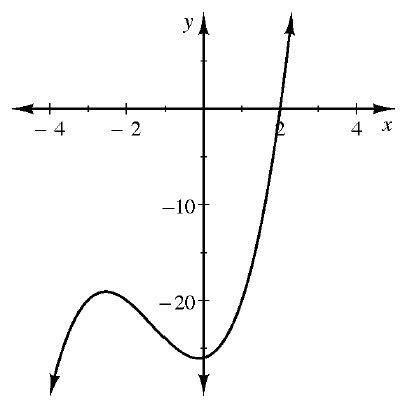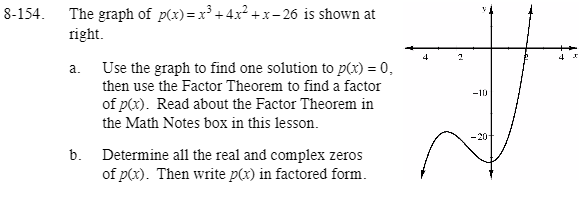Home > CCA2 > Chapter 8 > Lesson 8.3.2 > Problem8-154

8-154.
1.The graph of p(x) = x3 + 4x2 + x − 26 is shown at right. Homework Help ✎

1. Use the graph to find one solution to p(x) = 0, then use the Factor Theorem to find a factor of p(x). Read about the Factor Theorem in the Math Notes box in this lesson.

2. Determine all the real and complex zeros of p(x). Then write p(x) in factored form.Where does the graph cross the x-axis? What is the associated factor?

Divide the factor from part (a) into p(x) to find a quadratic factor.
Then use the Quadratic Formula to find the roots.
Use the roots to write the associated factors.